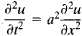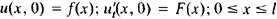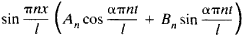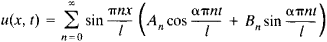# Fourier Method

The following article is from The Great Soviet Encyclopedia (1979). It might be outdated or ideologically biased.

## Fourier Method

a method of solution of partial differential equations by means of separation of variables. Proposed by J. Fourier as a tool for the solution of heat conduction problems, it was formulated in full generality by M. V. Ostrogradskii in 1828.

In the Fourier method a solution of an equation satisfying initial homogeneous and boundary conditions is sought in the form of a sum of solutions satisfying the boundary conditions, each of these solutions being a product of a function of the space variables by a function of time. To find such solutions we must first find the eigenvalues and eigenfunctions of certain differential operators and then obtain the corresponding eigenfunction expansions of the functions involved in the initial conditions.

In particular, the representation of functions in terms of Fourier series and Fourier integrals is made use of in the study of vibrations of a string and in the study of heat conduction in a rod. For example, the study of small-amplitude vibrations of a string of length I and with fixed endpoints reduces to the solution of the equationwith boundary conditions u(0, t) = u(l, t) = 0 and initial conditions. The solutions of the equation that have the form X(x)T(t) and satisfy the boundary conditions are given by the formulaFor a suitable choice of the coefficients An and Bn the functionis a solution of the problem.

V. A. Steklov solved many important problems involving the use of the Fourier method.

Mentioned in ?
References in periodicals archive ?
Tappert, "Applications of the split-step Fourier method to the numerical solution of nonlinear and variable coefficient wave equations," SIAM Review, vol.
In Section 4 we show the theoretical and implementation of Milstein scheme using the Fourier method. In the last section we give numerical example to the show the convergence behaviour.
We use the split-step Fourier method during the numerical solution of the equation, with the Fast Fourier Transform algorithm on the dispersive step [15,16].
Section 3 describes the numerical calculation of SC and DW generation for different birefringent FO profiles by using the split-step Fourier method  and the four-order Runge-Kutta algorithm .
Fromme and Leissa  extended the Fourier method to investigate the free vibration of the rectangular parallelepiped with simple classical boundary conditions based on the three-dimensional elasticity theory.
Three-dimensional Poisson equation for pressure is solved by Fourier method in combination with the tridiagonal matrix method (Thomas algorithm) that is applied to determine the Fourier coefficients [16, 18].
We can use the split-step Fourier method [11, 16-18] to solve the linear term of (1), while the nonlinear term can be described as follows:
Finally, we compare the efficacy of the new imaging method with the Direct Fourier Method.
Due to the fact that the phase-modified item with correct distance R is used in modified Fourier method, its imaging result (Fig.
Secondly, the proposed methods are proven to be unconditionally stable by using the Fourier method. Furthermore, the dispersion analyses are given.
The shape of the shell from the two populations of the bivalve were analyzed using the Elliptic Fourier method that allowed various simple transformations so that the results will be invariant to size, location, rotation, and starting point of the digitized outline .

Site: Follow: Share:
Open / Close